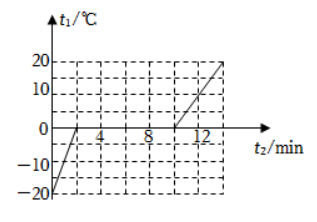【答案】 $8.4 \times 10^4 \mathrm{~J} ; 350 \mathrm{~W} ; 2.1 \times 10^3 \mathrm{~J} /\left(\mathrm{kg} \cdot{ }^{\circ} \mathrm{C}\right)$ 。
【分析】 (1) 由图象可知, 第 $10 \sim 14 \mathrm{~min}$ 内, 该物质处于液态, 根据 $Q_{\text {吸 }}=c_1 m \Delta t$ 可以求出吸收的热量, 然后根据 $P=\frac{Q \text { 吸可求出吸 }}{t}$ 热功率；
（ 2 ） 由图可知, 该物质在 $0 \sim 2 \min$ 时间内处于固态, 由于吸热功率恒定不变, 根据 $Q=P t$ 可求出这一段时间内吸收的热 量, 然后利用公式 $c=\frac{Q \text { 吸 }}{m \Delta t}$ 可以求出固态下的比热容。

【解答】解：（1）由图象可知, 第 $10 \sim 14 \mathrm{~min}$ 内, 该物质处于液态,

$$Q_{\text {吸 }}=\mathrm{cm} \Delta t=4200 \mathrm{~J} /\left(\mathrm{kg} \cdot{ }^{\circ} \mathrm{C}\right) \times 1 \mathrm{~kg} \times 20^{\circ} \mathrm{C}=8.4 \times 10^4 \mathrm{~J} \text {, }$$

$$t=4 \text { 分钟 }=240 \mathrm{~s},$$

$$P=\frac{Q \text { 吸 }}{t}=\frac{8.4 \times 10^4 \mathrm{~J}}{240 \mathrm{~s}}=350 \mathrm{~W} \text { ； }$$
（2） 物体在 $0 \sim 2 \min$ 时间内, 加热时间 $T=2 \min =120 \mathrm{~s}$, 物体吸收的热量:
$$Q_{\text {吸 }}{ }^{\prime}=P T=350 \mathrm{~W} \times 120 \mathrm{~s}=42000 \mathrm{~J},$$

$$c_1=\frac{Q_{\text {吸 }}}{m \Delta t_1}=\frac{42000 \mathrm{~J}}{1 \mathrm{~kg} \times 20^{\circ} \mathrm{C}}=2.1 \times 10^3 \mathrm{~J} /\left(\mathrm{kg} \cdot{ }^{\circ} \mathrm{C}\right) 。$$